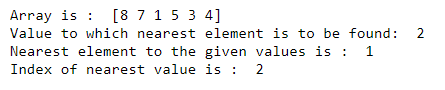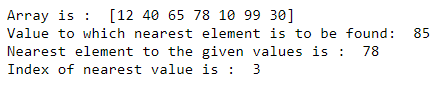# Find the nearest value and the index of NumPy Array

• Last Updated : 03 Mar, 2021

In this article, let’s discuss finding the nearest value and the index in an array with NumPy. Numpy provides a high-performance multidimensional array object, and tools for working with these arrays. We will make use of two of many functions provided by NumPy library to calculate the nearest value and the index in the array. Those two functions are numpy.abs() and numpy.argmin(). The approach to finding the nearest value and the index in the array is given below:

Approach:

Attention geek! Strengthen your foundations with the Python Programming Foundation Course and learn the basics.

To begin with, your interview preparations Enhance your Data Structures concepts with the Python DS Course. And to begin with your Machine Learning Journey, join the Machine Learning - Basic Level Course

• Take an array, say, arr[] and an element, say x to which we have to find the nearest value.
• Call the numpy.abs(d) function, with d as the difference between element of array and x, and store the values in a difference array, say difference_array[].
• The element, providing minimum difference will be the nearest to the specified value.
• Use numpy.argmin(), to obtain the index of the smallest element in difference_array[]. In the case of multiple minimum values, the first occurrence will be returned.
• Print the nearest element, and its index from the given array.

Let us look at below examples based on the above approach.

Example 1:

## Python3

 `import` `numpy as np`` ` ` ` `# array``arr ``=` `np.array([``8``, ``7``, ``1``, ``5``, ``3``, ``4``])``print``(``"Array is : "``, arr)`` ` `# element to which nearest value is to be found``x ``=` `2``print``(``"Value to which nearest element is to be found: "``, x)`` ` `# calculate the difference array``difference_array ``=` `np.absolute(arr``-``x)`` ` `# find the index of minimum element from the array``index ``=` `difference_array.argmin()``print``(``"Nearest element to the given values is : "``, arr[index])``print``(``"Index of nearest value is : "``, index)`

Output:In the above example, we have taken an array from which we need to find the nearest element to the specified value. The specified value is 2. We subtract the given value from each the element of the array and store the absolute value in a different array. The minimum absolute difference will correspond to the nearest value to the given number. In our example, (2-1) yield 1. Thus, the index of minimum absolute difference is 2 and the element from the original array at index 2 is 1. Thus, 1 is nearest to the given number i.e 2.

Example 2:

## Python3

 `import` `numpy as np`` ` ` ` `# array``arr ``=` `np.array([``12``, ``40``, ``65``, ``78``, ``10``, ``99``, ``30``])``print``(``"Array is : "``, arr)`` ` `# element to which nearest value is to be found``x ``=` `85``print``(``"Value to which nearest element is to be found: "``, x)`` ` `# calculate the difference array``difference_array ``=` `np.absolute(arr``-``x)`` ` `# find the index of minimum element from the array``index ``=` `difference_array.argmin()``print``(``"Nearest element to the given values is : "``, arr[index])``print``(``"Index of nearest value is : "``, index)`

Output:In the above example, we have taken an array from which we need to find the nearest element to the specified value. The specified value is 85. We subtract the given value from each the element of the array and store the absolute value in a different array. The minimum absolute difference will correspond to the nearest value to the given number. In the above example, (78-85) yields 7. Thus, the index of minimum absolute difference is 3 and the element from the original array at index 3 is 78. Thus, 78 is nearest to the given number i.e 85.

My Personal Notes arrow_drop_up